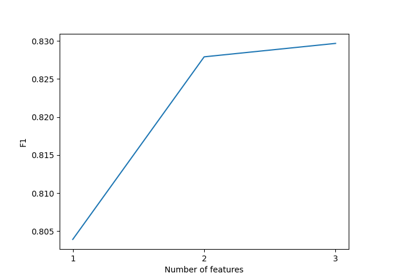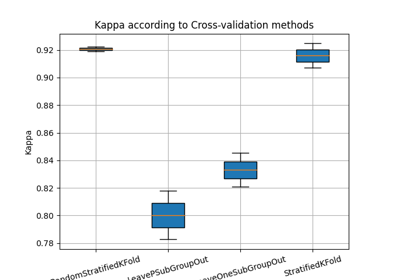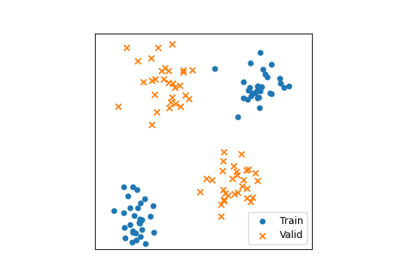# LeavePSubGroupOut¶

class `museotoolbox.cross_validation.``LeavePSubGroupOut`(valid_size=0.5, n_repeats=False, random_state=False, verbose=False)[source]

Generate a Cross-Validation using subgroup (each group belong to a unique label).

Parameters
• valid_size (float, default 0.5.) – From 0 to 1.

• n_repeats (int or bool, optional (default=False)) – If False, n_splits is 1/valid_size (default : 1/0.5 = 2). If int : will iterate the number of times given in n_splits.

• random_state (integer or None, optional (default=False)) – If int, random_state is the seed used by the random number generator; If None, the random number generator is created with `time.time()`.

• verbose (integer or False, optional (default=False)) – Controls the verbosity: the higher the value is, the more the messages are detailed.

Manage cross-validation methods to generate the duo valid/train samples.

Methods

 `__init__`([valid_size, n_repeats, …]) Manage cross-validation methods to generate the duo valid/train samples. `get_n_splits`([X, y, groups]) Returns the number of splitting iterations in the cross-validator. `save_to_vector`(vector, field[, group, …]) Save to vector files each fold from the cross-validation. `split`(X, y[, groups]) Split the vector/array according to y and groups.

## Examples using `museotoolbox.cross_validation.LeavePSubGroupOut`¶